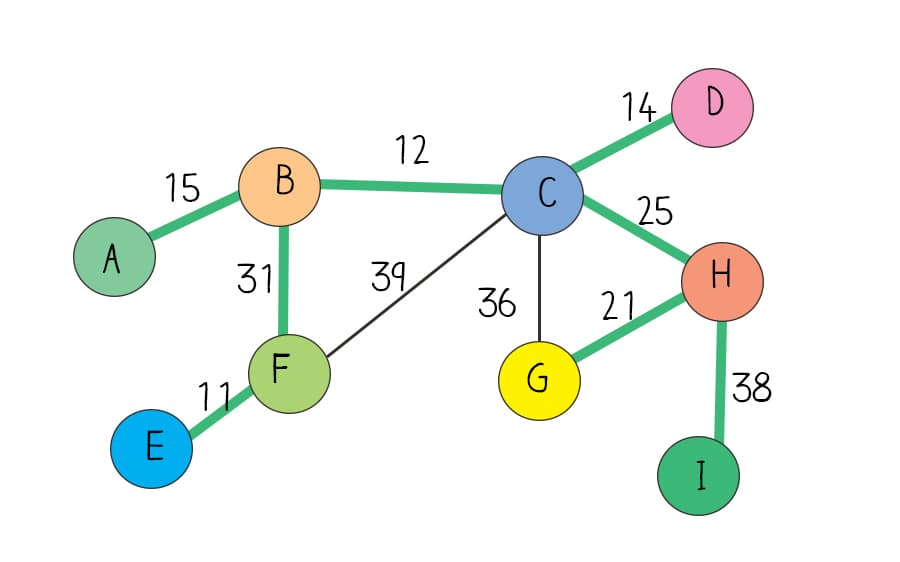A minimum spanning tree(MST) is a subset of a weighted undirected graph, in which edges that forms a tree (no cycle graph) includes every vertex in a graph, where the sum of the weights of all the edges in the tree is minimized. Kruskal’s algorithm is an algorithm to find MST in a weighted undirected graph using union find.### How Kruskal’s algorithm works?

Kruskal’s algorithm is a greedy algorithm. In the process, it always adds the next lowest weight edge. In order not to form a cycle, it uses find and union methods in the DisjointSet class. If two vertices of an edge don’t share the same root confirmed by find method, you add this edge to MST. After adding the edge, call union method to union this two vertices so that they will not be added again.

### Define an Edge classe

MST is a weighted graph problem. You can define edge as a class, in which there are source vertex, target vertex, and weight.

## Python

Next you construct a graph. A graph is often represented as adjacency list. Here a graph is simplified. You don’t need create hashmap to represent graph. Instead you define edges and vertices separately. edges is a list of GraphEdge objects. nodes is a set of individual vertices.

## Python

### Kruskal’s algorithm

Now you can apply Kruskal’s algorithm. Since it uses Disjoint set data structure’s union and find method, you can create an object of DisjointSet class, which has been introduced in Disjoint set and union find post. Call makeSet method with nodes to establish a disjoint set. Sort the list of edges by their weights in ascending order. Start from the smallest-weight edge, if the source node and target node are not in the same subset of a disjoint set, they is no cycle when adding them. You can add this edge to MST. Then you union these two nodes so that they will not be selected again.

The time complexity of Kruskal’s algorithm is O(E*logE) due to sorting, E is number of edges in the graph. Since find uses recursion, the space is required for recursive function’s call stacks. The Space complexity is  O(E*a(E+V)), where α(n) is the extremely slow-growing inverse Ackermann function due to optimization of path compression and union by rank in disjoint set implementation.